# 06-240/Classnotes For Tuesday October 10

## A Quick Summary by Dror

(Intentionally terse. A sea of details appears in the book and already appeared on the blackboard. But these are useless without some organizing principles; in some sense, "understanding" is precisely being able to see those principles within the sea of details. Yet don't fool yourself into thinking that the principles are enough even without the details!)

Theorem. A finite generating set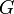$G$ has a subset which is a basis.

Proof Sketch. Grab more and more elements of$G$ so long as they are linearly independent. When you can't any more, you have a basis.

Lemma. (The Replacement Lemma) If$G$ generates and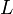$L$ is linearly independent, then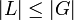$|L|\leq|G|$ and you can replace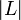$|L|$ of the elements of$G$ by the elements of$L$, and still have a generating set.

Proof Sketch. Insert the elements of$L$ one by one, and for each one that comes in, take one out of$G$. Which one? One used in expressing the newcomer in terms of the vectors already in$G$. Such a vector must exist or else the newcomer is a linear combination of some of the elements of$L$, but$L$ is linearly independent.

Theorem. If a vector space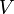$V$ has a finite basis, all bases thereof are finite and have the same number of elements, the "dimension of$V$".

Proof Sketch. By replacement,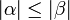$|\alpha|\leq|\beta|$ and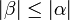$|\beta|\leq|\alpha|$.

Theorem. Assume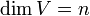$\dim V=n$.

1. If$G$ generates,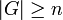$|G|\geq n$. In case of equality,$G$ is a basis.
2. If$L$ is linearly independent,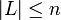$|L|\leq n$. In case of equality,$L$ is a basis.

Proof Sketch.

1. Find a basis within$G$; it has$n$ elements.
2. Use replacement to place the elements of$L$ within some basis.